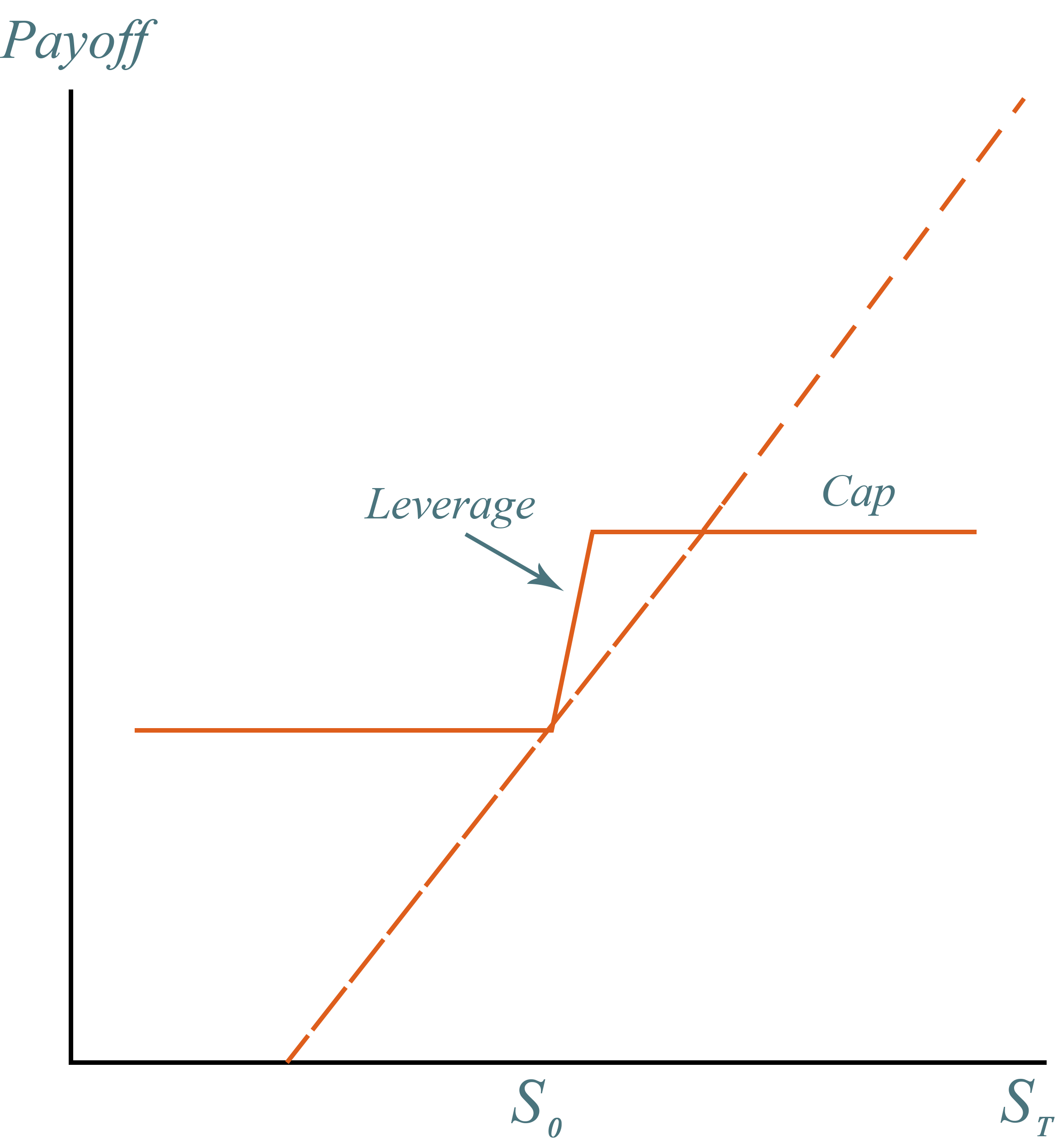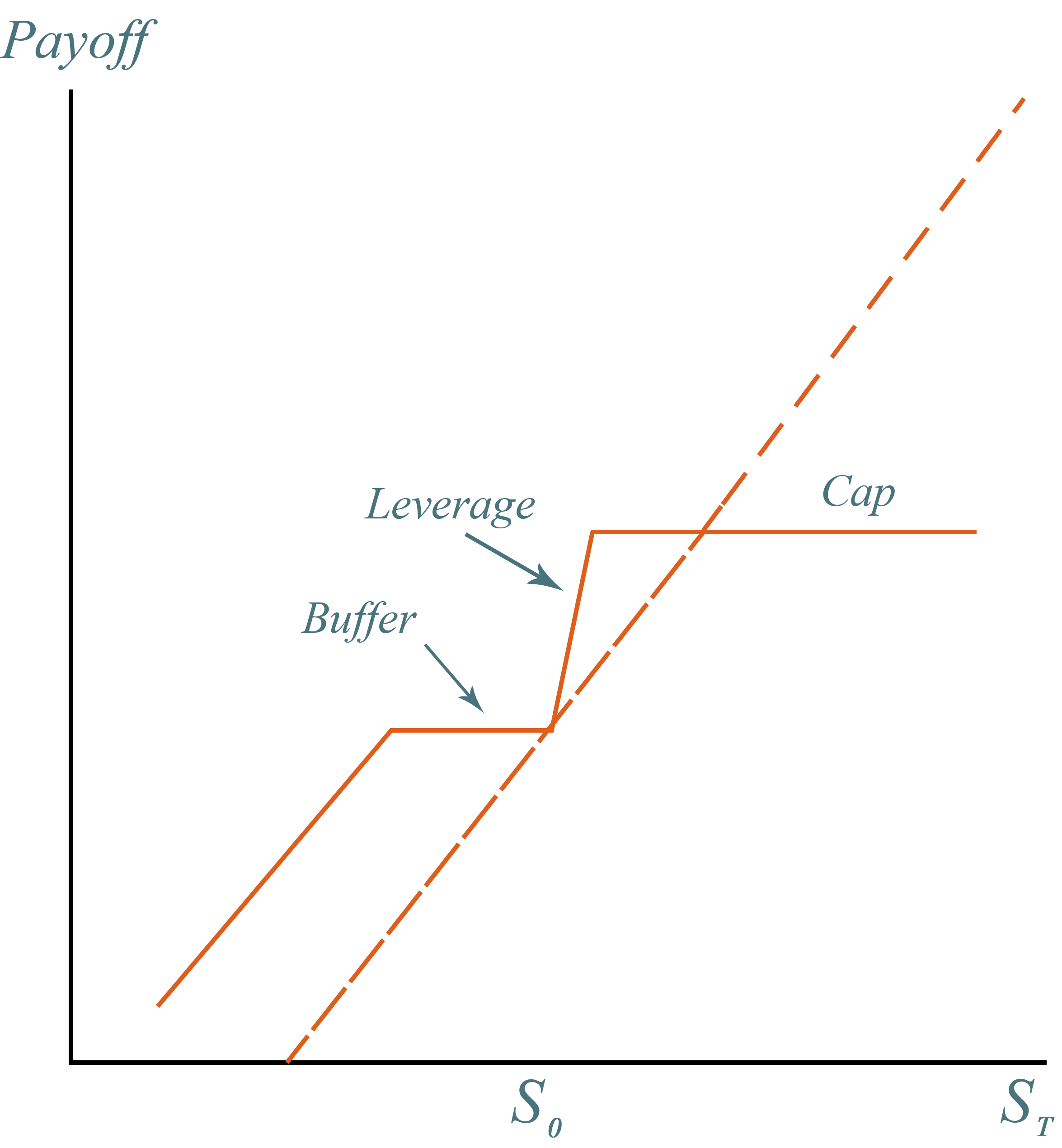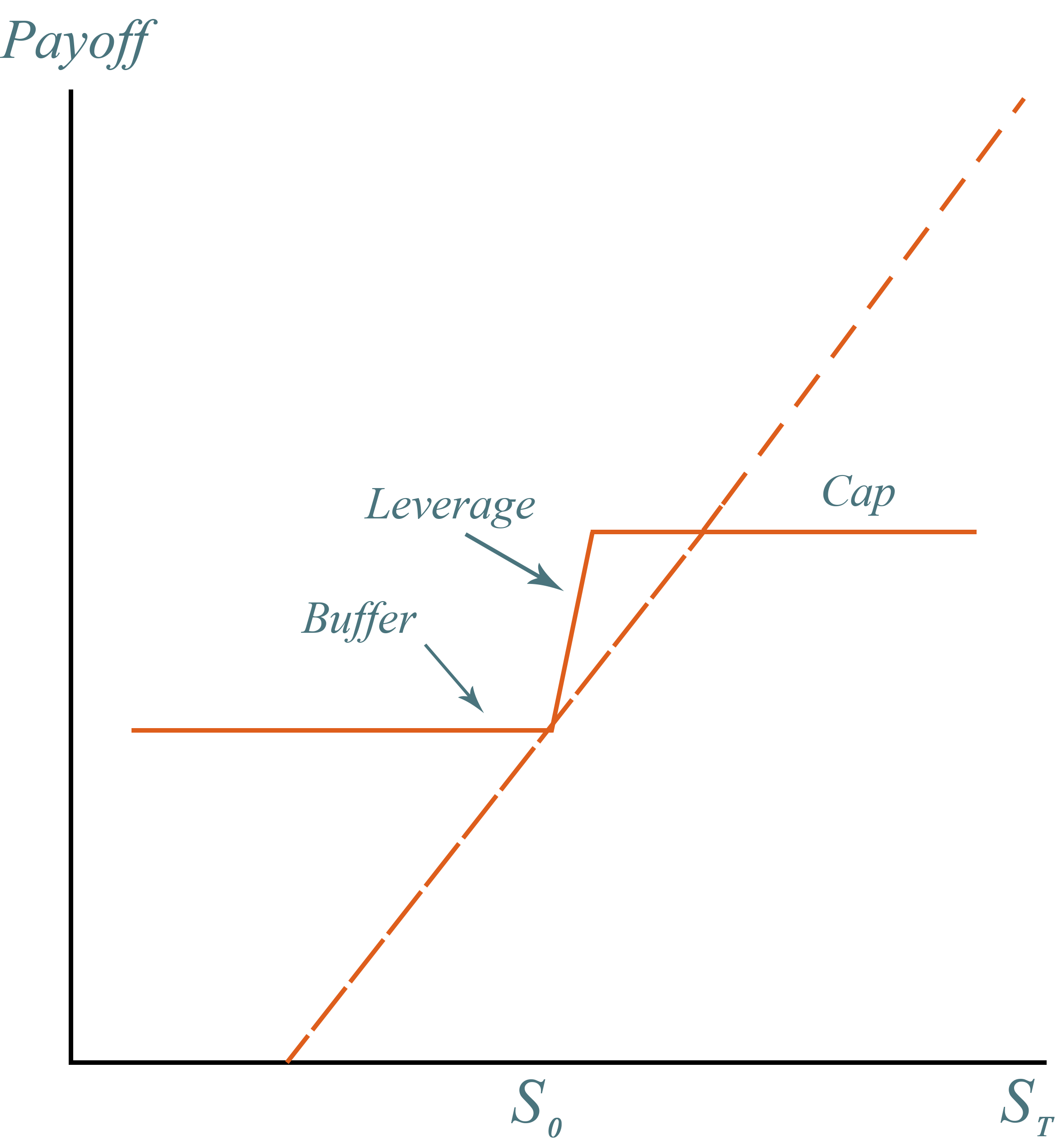Tools

# Structured Product Calculators

Made By: SLCG Economic Consulting Staff

## PLUS® Calculator

### (Return Optimization Securities/Stock Market Upturn Notes)

PLUS®, Return Optimization Securities, and Stock Market Upturn Notes Value are structured products with the same type of payoff. These products expose investors to the linked security's downside risk while offering levered but generally limited upside potential. They do not make coupon payments or promise any interest. Some varieties also have a capped return.

The following example is based on a Morgan Stanley PLUS, assuming that the risk free rate is 5%, the implied volatility of the underlying stock is 25%, the dividend yield of the underlying stock is 1% and the credit default swap ("CDS") spread of the issuer (Morgan Stanley) is 1%. The contract has a maturity of 1 year. The PLUS has a leverage ratio of 2.5 and a cap rate of 20%. We assume that the initial investment is $100.### Calculation Table Enter your inputs below to determine your Product Value: ## Buffered PLUS® Calculator ### (Partial Protection Return Optimized Securities / Equity Buffer Notes / Leveraged Equity Index-Linked Notes) Buffered PLUS®, Partial Protection Return Optimization Securities, Equity Buffered Notes, and Leverage Equity Index-Linked Notes expose investors to most of the linked security's downside risk while offering levered but generally limited upside potential. These products do not make coupon payments or promise any interest. Some varieties have a capped return. The following example is based on a Morgan Stanley Buffered PLUS, assuming that the risk free rate is 5%, the implied volatility is 25%, the dividend yield of the underlying stock is 1%, and the credit default swap ("CDS") spread of the issuer is 1%. The product has a two-year maturity, a leverage ratio of 2.5, a cap rate of 20% and a 15% loss buffer. We assume that the initial investment is$100.## Principal Protected Notes Calculator

Principal Protected Notes ("PPN") are a general class of structured products that 'guarantee' the investor's principal. Principal protection is also a feature applied to several other types of products, such as Absolute Return Barrier Notes. Principal protected notes provide a levered return if the linked security has a positive return, while limiting potential losses to zero. Some PPNs have a capped return.

The following PPN valuation example assumes the risk free rate is 5%, the implied volatility of the underlying stock is 25%, the dividend yield of the underlying stock is 1%, and the credit default swap ("CDS") spread of the issuer is 1%. The note has a two-year maturity, a leverage ratio of 2.5, and a cap rate of 20%. We assume that the initial investment is \$100.